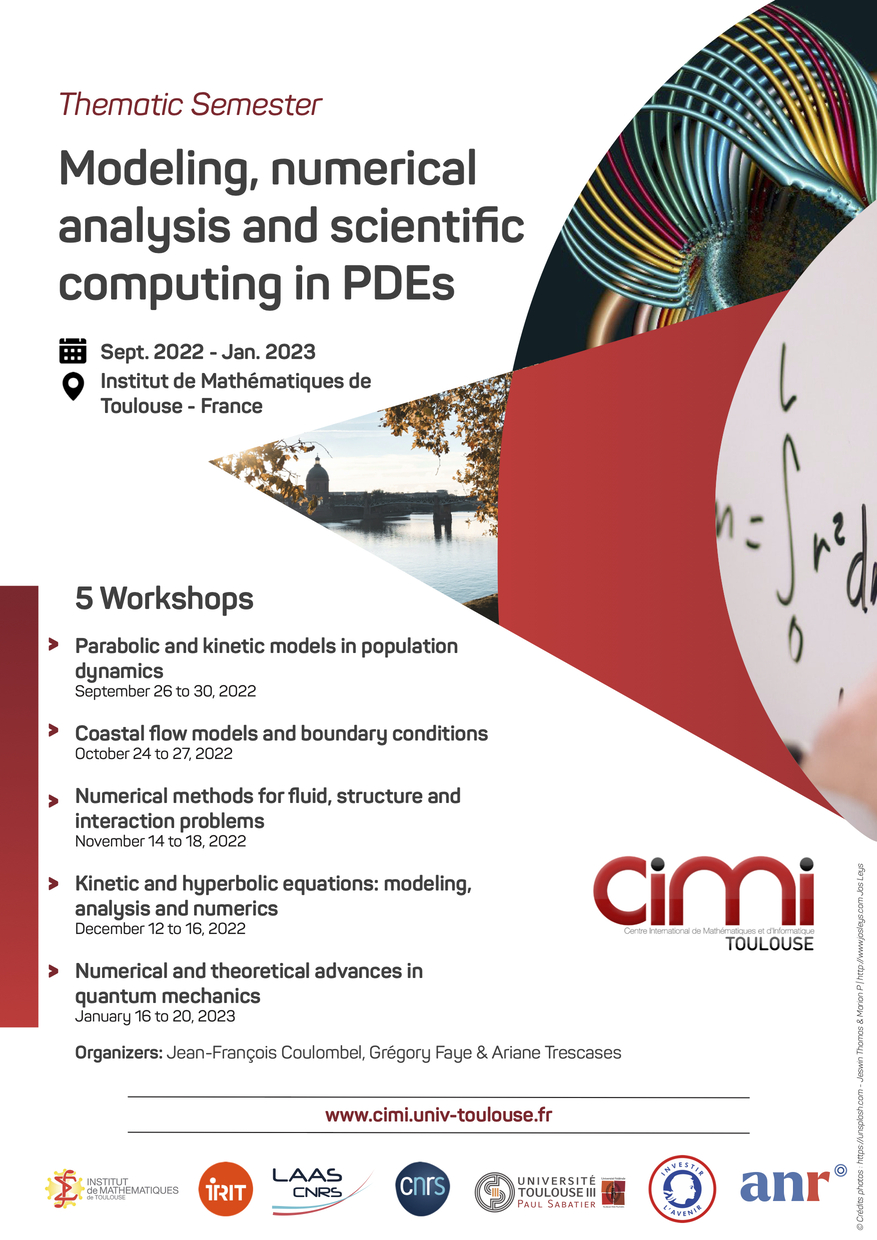#Modeling, numerical analysis and scientific computing in PDEs

September 1, 2022 to January 31, 2023
IMT
Europe/Paris timezone

### Contact:

The modelling and understanding of physical and natural phenomena is an important field of research in the applied mathematical community. The models of interest arise in the physical sciences, in biology, engineering, etc. In many cases, a phenomenon of interest is modeled by some (system of) partial differential equations and the main goals of the mathematical community are to understand how the solutions to these equations, when shown to exist, can be approximated by algorithms that are efficiently implemented on computers. Such questions as stability issues, rigorous justifications of asymptotic behaviors, or convergence of numerical algorithms have been much developed for decades.

This semester aims at exploring up to date questions in this field, which are of interest for researchers in mathematics and related fields (fluid dynamics, quantum mechanics, ecology, biology and so on) with a balance between the derivation and theoretical analysis of models, the construction of efficient (possibly high order) numerical methods and their practical implementation on a computer.

Workshops:

Parabolic and kinetic models in population dynamics, 26 sept. - 30 sept. 2022Starts
Ends
Europe/Paris
IMT
Amphithéâtre Schwartz